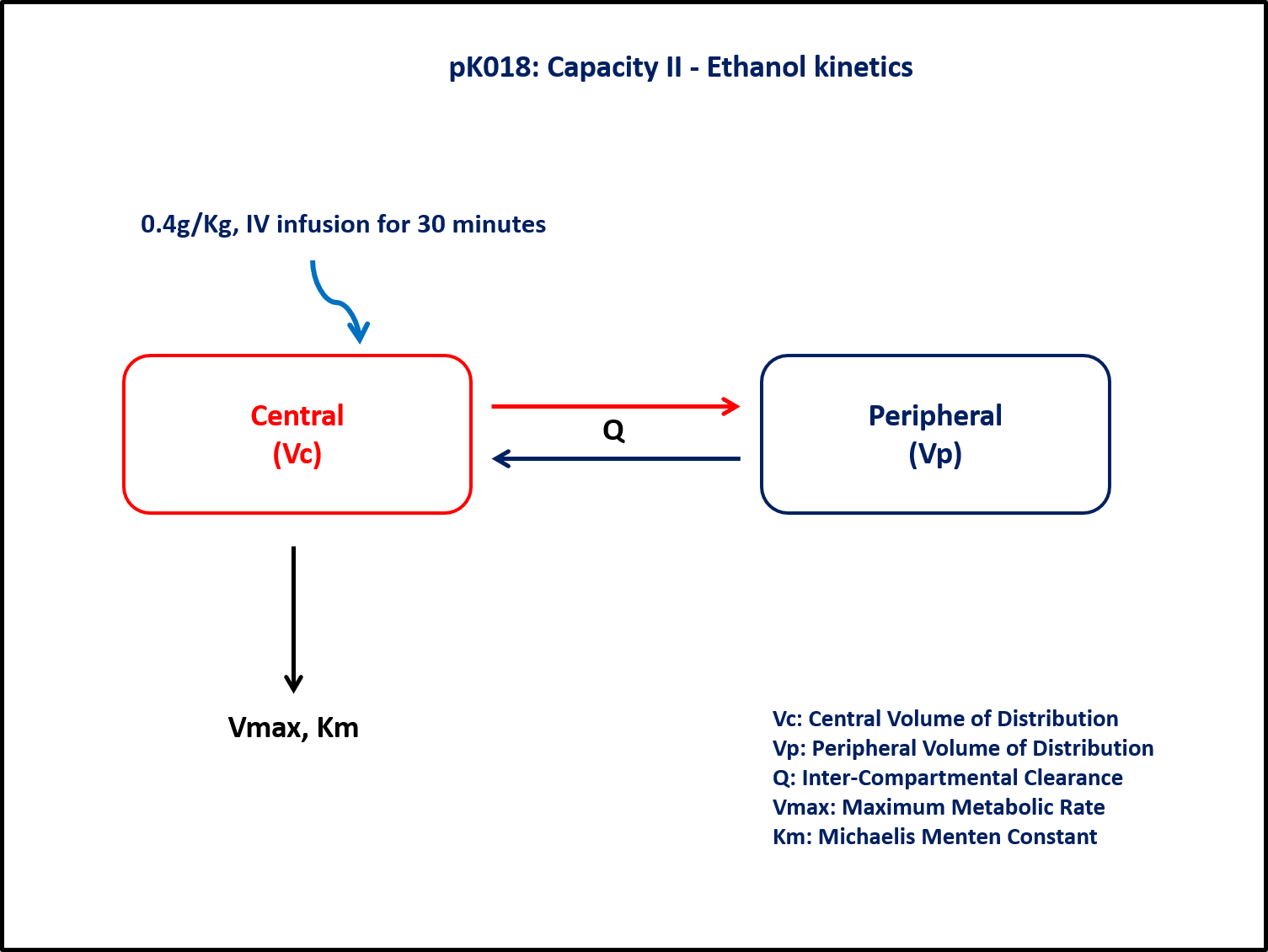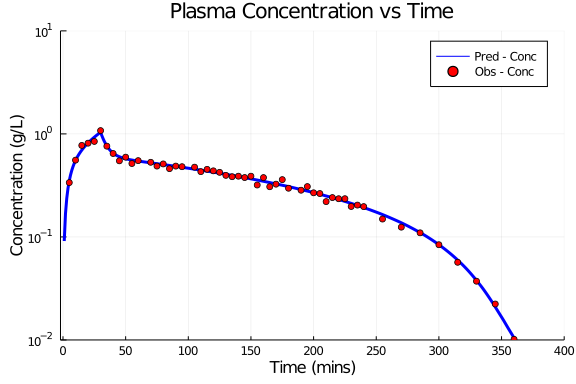# Exercise PK18 - Capacity II - Ethanol kinetics

### Background

• Structural model - Two compartment disposition model with nonlinear elimination

• Route of administration - IV infusion

• Dosage Regimen - 0.4g/Kg (i.e.,28g for a 70Kg healthy individual), infused over a time span of 30 minutes

• Number of Subjects - 1### Learning Outcome

In this model, you will learn -

• To build Two compartment disposition model, the drug is given as Intravenous Infusion which follows Michaelis Menten Kinetics.

• To estimate the fundamental parameters involved in building the model.

• To apply differential equation in the model as per the compartment model.

• To design the dosage regimen for the subjects and simulate the plot.

### Objectives

In this tutorial, you will learn how to build Two Compartment disposition model with Non-linear elimination following Intravenous infusion and simulate the model for single subject and single dosage regimen.

### Libraries

call the "necessary" libraries to get started

using Pumas
using CSV
using Plots
using StatsPlots
using Random


### Model

In this two compartment model, we administer dose on Central compartment.

pk_18           = @model  begin
@param begin
tvvmax      ∈ RealDomain(lower=0)
tvkm        ∈ RealDomain(lower=0)
tvQ         ∈ RealDomain(lower=0)
tvvc        ∈ RealDomain(lower=0)
tvvp        ∈ RealDomain(lower=0)
Ω           ∈ PDiagDomain(5)
σ²_prop     ∈ RealDomain(lower=0)
end

@random begin
η           ~ MvNormal(Ω)
end

@pre begin
Vmax        = tvvmax * exp(η)
Km          = tvkm * exp(η)
Q           = tvQ * exp(η)
Vc          = tvvc * exp(η)
Vp          = tvvp * exp(η)
end

@dynamics begin
Central'    = - (Vmax/(Km+(Central/Vc))) * Central/Vc + (Q/Vp) * Peripheral - (Q/Vc) * Central
Peripheral' = - (Q/Vp) * Peripheral + (Q/Vc) * Central
end

@derived begin
cp         = @. Central/Vc
dv         ~ @. Normal(cp, sqrt(cp^2*σ²_prop))
end
end

PumasModel
Parameters: tvvmax, tvkm, tvQ, tvvc, tvvp, Ω, σ²_prop
Random effects: η
Covariates:
Dynamical variables: Central, Peripheral
Derived: cp, dv
Observed: cp, dv


### Parameters

The parameters are as given below. tv represents the typical value for parameters.

• Vmax - Maximum rate of elimination (mg/hr)

• Km - Michaelis-Menten rate constant (mg/L)

• Q - Intercompartmental Clearance (L/hr)

• Vc - Volume of Central Compartment (L)

• Vp - Volume of Peripheral Compartment (L)

• Ω - Between Subject Variability

• σ - Residual error

param  = (tvvmax     = 0.0812189,
tvkm       = 0.0125445,
tvQ        = 1.29034,
tvvc       = 8.93016,
tvvp       = 31.1174,
Ω          = Diagonal([0.0,0.0,0.0,0.0,0.0]),
σ²_prop    = 0.005)

(tvvmax = 0.0812189, tvkm = 0.0125445, tvQ = 1.29034, tvvc = 8.93016, tvvp
= 31.1174, Ω = [0.0 0.0 … 0.0 0.0; 0.0 0.0 … 0.0 0.0; … ; 0.0 0.0 … 0.0 0.0
; 0.0 0.0 … 0.0 0.0], σ²_prop = 0.005)


### Dosage Regimen

0.4g/Kg (i.e.,28g for a 70Kg healthy individual), infused over a time span of 30 minutes, given to a single subject.

ev1   = DosageRegimen(28,time=0, cmt=1, duration=30)
sub1  = Subject(id=1,events=ev1)

Subject
ID: 1
Events: 2


### Simulation

Lets simulate for plasma concentration with the specific observation time points after Intravenous administration.

Random.seed!(1234)
sim_sub = simobs(pk_18,sub1,param,obstimes=0:1:360)
df1     = DataFrame(sim_sub)
dropmissing!(df1, :cp)
delete!(df1, )


### DataFrame & Plot

Use the DataFrame for Plotting where

• cp - Predicted concentration

• dv - Observed concentration

df1_dv = filter(x -> x.time in [5,10,15,20,25,30,35,40,45,50,55,60,70,75,80,85,90,95,105,110,
115,120,125,130,135,140,145,150,155,160,165,170,175,180,190,195,200,205,210,215,220,225,230,
235,240,255,270,285,300,315,330,345,360], df1)

@df df1 plot(:time, :cp, yaxis=:log,
label= "Pred - Conc", xlabel="Time (mins)", ylabel="Concentration (g/L)",
linewidth=3, color=[:blue], title="Plasma Concentration vs Time",
xlims=(-2,400), xticks=[0,50,100,150,200,250,300,350,400],
yticks=[0.01,0.1,1,10], ylims=(0.01,10))
@df df1_dv scatter!(:time, :dv, label="Obs - Conc", color=[:red])